# 运动学¶

## 加速¶

Klipper总使用常加速度策略——打印头的速度总是梯度变化到新的速度，而非使用速度突变的方式。Klipper着眼于打印件和打印头之间的速度变化。离开挤出机的耗材十分脆弱，突然的移动速度和/或挤出流量突变可能会导致造成打印质量或床黏着能力的下降。甚至在无挤出时，如果打印头和打印件顶端在同一水平面时，喷嘴的速度突变有可能对刚挤出的耗材进行剐蹭。限制打印头相对于打印件的速度，可以减少剐蹭打印件的风险。

Klipper实现恒加速度控制，关键的方程如下：

``````velocity(time) = start_velocity + accel*time
``````

## 梯形发生器¶

Klipper 使用传统的"梯形发生器"来产生每个动作的运动--每个动作都有一个起始速度，先恒定的加速度加速到一个巡航速度，再以恒定的速度巡航，最后用恒定的加速度减速到终点速度。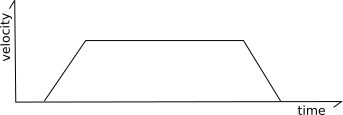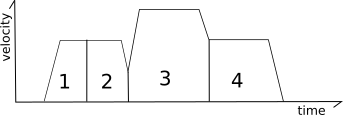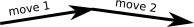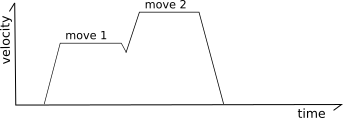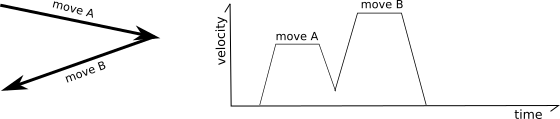``````end_velocity^2 = start_velocity^2 + 2*accel*move_distance
``````

### 预计算结果平滑¶

Klipper 实现了一种用于平滑短距离之字形移动的机制。参考以下移动：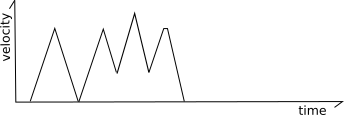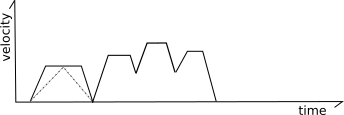## 生成步数（Generating steps）¶

Klipper使用一个迭代求解器来生成每个步进的步进时间。该代码包含了计算打印头在每个时间点上的理想笛卡尔坐标的公式，它还有运动学公式来计算基于这些笛卡尔坐标的理想步进位置。通过这些公式，Klipper可以确定步进电机在每个步进位置时的理想步进时间。然后在这些计算出的时间内安排给定的步进。

``````move_distance = (start_velocity + .5 * accel * move_time) * move_time
``````

``````move_distance = cruise_velocity * move_time
``````

``````cartesian_x_position = start_x + move_distance * total_x_movement / total_movement
cartesian_y_position = start_y + move_distance * total_y_movement / total_movement
cartesian_z_position = start_z + move_distance * total_z_movement / total_movement
``````

### 笛卡尔机器¶

``````stepper_x_position = cartesian_x_position
stepper_y_position = cartesian_y_position
stepper_z_position = cartesian_z_position
``````

### CoreXY 机器¶

``````stepper_a_position = cartesian_x_position + cartesian_y_position
stepper_b_position = cartesian_x_position - cartesian_y_position
stepper_z_position = cartesian_z_position
``````

### 三角洲机器¶

``````stepper_position = (sqrt(arm_length^2

- (cartesian_x_position - tower_x_position)^2
- (cartesian_y_position - tower_y_position)^2)
+ cartesian_z_position)
``````

### 挤出机运动学¶

Klipper 在自身的运动学类中实现了挤出机的运动。由于每个打印头运动的时间和速度是完全已知的，因此可以独立于打印头运动的步长计算来计算挤出机的步长。

``````stepper_position = requested_e_position
``````

### 压力提前¶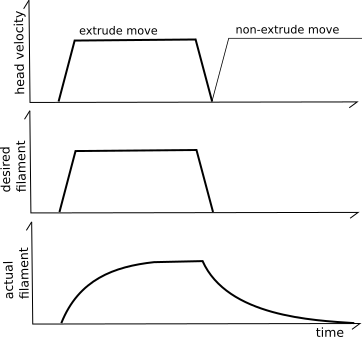"压力推进"系统试图通过使用一个不同的挤出机模型来解决这个问题。它不理想的假设送入挤出机的每mm^3耗材将导致该体积的mm^3立即被挤出，而是使用基于压力的模型。当耗材被推入挤出机时，压力会增加（如胡克定律），而挤出所需的压力则由通过喷嘴孔口的流速决定（如泊伊维尔定律）。关键的想法是，耗材、压力和流速之间的关系可以用一个线性系数来建模：

``````pa_position = nominal_position + pressure_advance_coefficient * nominal_velocity
``````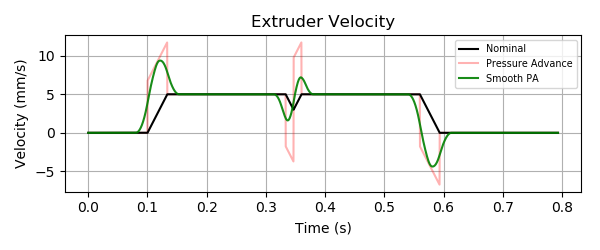“平滑”由挤出机位置在一小段时间内的加权平均值实现的（由 `pressure_advance_smooth_time` 配置参数指定）。这种平均可以跨越多个 g 代码移动。请注意，挤出机电机将如何在第一次挤出运动的标称起点之前开始移动，并在最后一次挤出运动的标称结束之后继续移动。

"平滑压力提前"的关键公式：

``````smooth_pa_position(t) =
( definitive_integral(pa_position(x) * (smooth_time/2 - abs(t - x)) * dx,
from=t-smooth_time/2, to=t+smooth_time/2)
/ (smooth_time/2)^2 )
``````